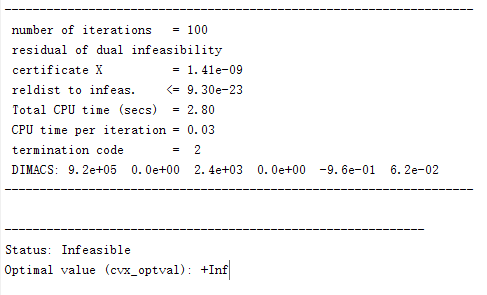# Can quad_over_lin solve this problem?

How to deal with the subject function X^3/Y^2, where X and Y are variables.

Presuming `X >= 0, Y > 0`, here is my CVX implementation of the formulation produced by @Michal_Adamaszek earlier in that thread.

Thank you for your help! I have noticed it, but i don’t know how to use it in subject function.

Do you understand it now, or do you know what you need to do?

my `z` is your `X`
my `y` is your `Y`
my `x` is a new variable which you can name what you want
my `s` can be your `s`.

Thanks! I know the relationship between the variables in that link and my variables, but i still don’t know how to write the cvx code here,i.e., ‘minimize (X^3/Y^2)’.

In my link, I said to use my x in place of z^3/y^2, so that would be minimize(x). Now just change the variable names to your code.

Thanks! I have dealt with this problem.But i have a new question that the first few times the program runs the results are ‘solved’ but suddenly the following results appear: Status: Infeasible
Optimal value (cvx_optval): +Inf. I want to know why this happened.We have no idea what you ran, to include the relation between the various runs you made. Are you using SCA - if so, read my posts on this forum about it.

Yes, I used SCA, i want to know which posts may help me. Thanks!